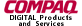gluPartialDisk.3glOpenGL man pages

```

```

## Name

```  gluPartialDisk - draw	an arc of a disk

```

## C Specification

```  void gluPartialDisk( GLUquadricObj *qobj,
GLint slices,
GLint loops,
GLdouble	startAngle,
GLdouble	sweepAngle )

```

## Parameters

```

innerRadius  Specifies the inner radius of the partial disk (can be zero).

slices       Specfies	the number of subdivisions around the z	axis.

loops	       Specifies the number of concentric rings	about the origin into
which the partial disk is subdivided.

startAngle   Specifies the starting angle, in	degrees, of the	disk portion.

sweepAngle   Specifies the sweep angle, in degrees, of the disk portion.

```

## Description

```  gluPartialDisk renders a partial disk	on the z=0 plane.  A partial disk is
similar to a full disk, except that only the subset of the disk from
startAngle through startAngle	+ sweepAngle is	included (where	0 degrees is
along	the +y axis, 90	degrees	along the +x axis, 180 along the -y axis, and
270 along the	-x axis).

The partial disk has a radius	of outerRadius,	and contains a concentric
no hole is generated.	 The partial disk is subdivided	around the z axis
into slices (like pizza slices), and also about the z	axis into rings	(as
specified by slices and loops, respectively).

With respect to orientation, the +z side of the partial disk is considered
to be	outside	(see gluQuadricOrientation). This means	that if	the
orientation is set to	GLU_OUTSIDE, then any normals generated	point along
the +z axis.	Otherwise, they	point along the	-z axis.

If texturing is turned on (with gluQuadricTexture), texture coordinates are
generated linearly such that where r=outerRadius, the	value at (r, 0,	0) is
(1, 0.5), at (0, r, 0) it is (0.5, 1), at (-r, 0, 0) it is (0, 0.5), and at
(0, -r, 0) it	is (0.5, 0).

```

```  gluCylinder, gluDisk,	gluNewQuadric, gluQuadricOrientation,